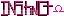-=+=- -=+=- -=+=- -=+=- -=+=- -=+=- -=+=- -=+=- -=+=- -=+=- -=+=- -=+=- -=+=- -=+=- -=+=- -=+=- -=+=- -=+=- -=+=- -=+=- -=+=- -=+=- -=+=- -=+=- -=+=- -=+=- -=+=- -=+=- -=+=- -=+=- (c) WidthPadding Industries 1987 0|697|0 -=+=- -=+=- -=+=- -=+=- -=+=- -=+=- -=+=- -=+=- -=+=- -=+=- -=+=- -=+=- -=+=- -=+=- -=+=- -=+=- -=+=- -=+=- -=+=- -=+=- -=+=- -=+=- -=+=- -=+=- -=+=- -=+=- -=+=- -=+=- -=+=- -=+=-
SoCoder -> Link Home -> Misc

HoboBenCreated : 10 June 2010

### Rounding - different tie-breaking methods

https://en.wikipedia.org/wiki/Rounding#Tie-breaking
I didn't realise there was more than one method. I quite like Banker's rounding, and I thought the section on Dithering was especially useful.

Friday, 11 June 2010, 03:06
JayenkaiI'm not sure about their analysis of the Round-up theory..
They suggest that .5's rounding up causes a slight imbalance, because 1 too many are rounded up..

But surely for every .5 there's an equal .0
Where .5 goes up, .0 stays down

That evens it out, right!?

Think of it in monetary terms.
1.00 -> 1.49 = 50 possible values always become £1
1.50 -> 1.99 = 50 possible values always become £2Saturday, 12 June 2010, 15:22
SchererererTechnically though, if even if you arbitrarily picked .2 as the magic number for rounding up, there's the same number of values between [0.0,0.2) as there is in the interval [.2,1.0), therefore any number chosen is theoretically not unbalanced.

Intuitively you could even consider all the numbers below zero (e.g. there's all the numbers [.2,1.0) u [1.0,0.2) which round to 1.0).